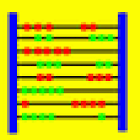Abakus-Diff

All Android applications categories

All Android games categories# Abakus-Diff

by: 2 9

9 Users
rating

## Screenshots

Description

Abakus is a computer algebra program which performs not only
arithmetic but even symbolic calculations of mathematical formulas.

The current Android version 1.0 contains the following functions:
- Exact arithmetic calculations, e.g. handling of integer numbers with "arbitrary" size
- Transforming mathematical formulas, e.g. factorization of polynomials
- Discussing mathematical functions, e.g. integration including solution steps
- Solving mathematical equations
- Vector and matrix calculations

Abakus-Diff is a free version of Abakus with reduced functionality:
You can calculate derivatives of mathematical functions including solution steps.

For example, the command "differentiate + trace" applied to the formula
"x*sin(x)" with variable "x" yields the following output:

diff(x*sin(x),x)
# Differentiate product: (f*g)' = f'*g+f*g'
diff(x,x)*sin(x)+x*diff(sin(x),x)
# diff(x,x) = 1
1*sin(x)+x*diff(sin(x),x)
# Table
1*sin(x)+x*cos(x)
# Simplify
sin(x)+x*cos(x).

Moreover Abakus contains a special editor for mathematical formulas.
So you can enter even complex expressions very fast.

At the moment, the following functions are supported:
sin, cos, tan, cot (trigonometric functions),
arcsin, arccos, arctan, arccot (inverse trigonometric functions),
sinh, cosh, tanh, coth (hyperbolic functions),
arsinh, arcosh, artanh, arcoth (inverse hyperbolic functions),
sqrt (square root),
crt (cubic root),
exp (exponential function),
ln (natural logarithm),
abs (Betrags function),
sgn (signum function).

Feasible operators are '+' (addition), '-' (subtraction),
'*' (multiplication), '/' (division), and '^' or '**' (power).

Tags: program to find logarithm , diff , abaku , abakus , abakus apk , abakus erhard apk , abakü , computer algebra , arctan 1.0135

from 2 reviews

"Awesome"

9